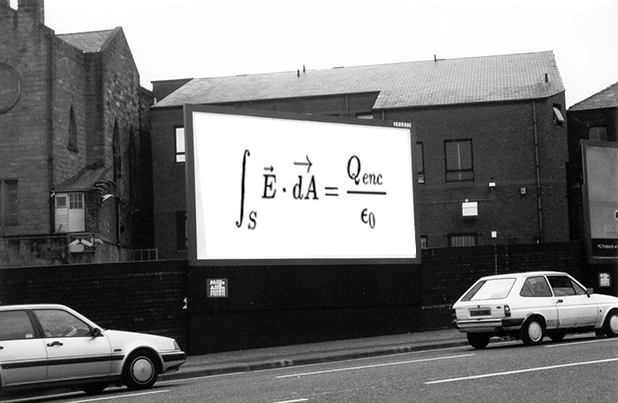# Integration over surfacesGauss' law is a very powerful method to determine the electric field due to a distribution of charges. The mathematical expression for Gauss' law is:

$\int_{S} \vec{E} \cdot \vec{dA}=\frac{Q_{enc}}{\epsilon_0}$

where $S$ is a surface, $\vec{E}$ is the electric field vector, $\vec{dA}$ is the infinitesimal area element, $Q_{enc}$ is the charge enclosed by $S$ and $\epsilon_0$ is a constant.

We now turn to the notion of integration over a specified surface. One can think about definite integration over a line interval, i.e. $\int_a^b f(x) dx$, as the area under the curve defined by $f(x)$. Similarly, the integral over a surface of a function can be thought of as the volume under the 2-d graph of the function. Using this definition, what is the integral

$\int_S x dA$

where the surface $S$ is the square in the $xy$-plane with corners at $(0,0), (1,0),(0,1),(1,1)$.

This problem is part of David's set on Gauss' Law.

×